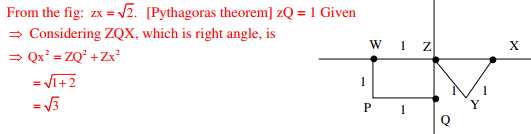# Geomatics Engineering - Online Test

Q1. The number of spectral bands in the Enhanced Thematic Mapper sensor on the remote sensing satellite Landsat-7 is
Explaination / Solution:
No Explaination.

Q2. The observed bearings of a traverse are given below:The stations(s) most likely to be affected by the local attraction is/are
Explaination / Solution:So, local attraction at only R

Q3. Consider the following sentences: All benches are beds. No bed is a bulb. Some bulbs are lamps. Which of the following can be inferred? i. Some beds are lamps. ii. Some lamps are beds.
Explaination / Solution:Since there is no direct relation given between lamps and beds. So, neither will be correct

Q4. __________ with someone else’s email account is now very serious offence.
Explaination / Solution:
No Explaination.

Q5. The minimum number of satellites needed for a GPS to determine its position precisely is
Explaination / Solution:
No Explaination.

Q6. The system that uses the Sun as a source of electromagnetic energy and records the naturally radiated and reflected energy from the object is called
Explaination / Solution:
No Explaination.

Q7. The staff reading taken on a workshop floor using a level is 0.645 m. The inverted staff reading taken to the bottom of a beam is 2.960 m. The reduced level of the floor is 40.500 m. The reduced level (expressed in m) of the bottom of the beam is
Explaination / Solution:

RL of bottom of beam = 40.5+2.96+0.645 = 44.105m

Q8. The magnitudes of vectors P, Q and R are 100 kN, 250 kN and 150 kN, respectively as shown in the figure.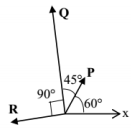The respective values of the magnitude (in kN) and the direction (with respect to the x-axis) of the resultant vector are
Explaination / Solution:

Resolving components w.r.t x-axis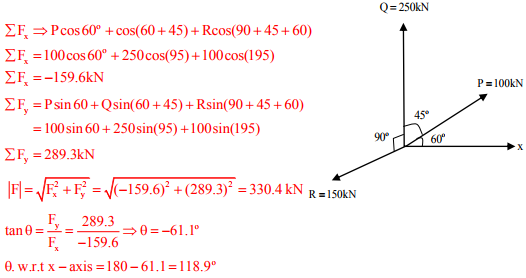Q9. The bearings of two inaccessible stations, S1 (Easting 500 m, Northing 500 m) and S2 (Easting 600 m, Northing 450 m) from a station S3 were observed as 225° and 153° 26′ respectively. The independent Easting (in m) of station S3 is:
Explaination / Solution: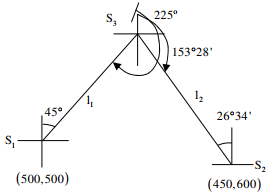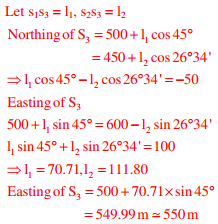Q10. X is 1 km northeast of Y. Y is 1 km southeast of Z. W is 1 km west of Z. P is 1 km south of W. Q is 1 km east of P. What is the distance between X and Q in km?
Explaination / Solution: